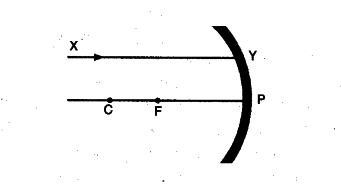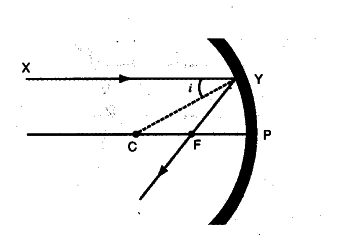# A ray of light XY is incident on a mirror as shown in the figure

A ray of light XY is incident on a mirror as shown in the figure. The angle of incidence for this ray is the angle between it and the line joining two other points in the figure. Name these two points and show the course of rays after reflection.The angle of incidence is the angle between the incident ray and the normal at the point of incidence. A line joining the centre of curvature and the point of incidence is a normal at the point of incidence. Thus, the given two points are C and Y as shown in figure: The course of rays is as shown below.